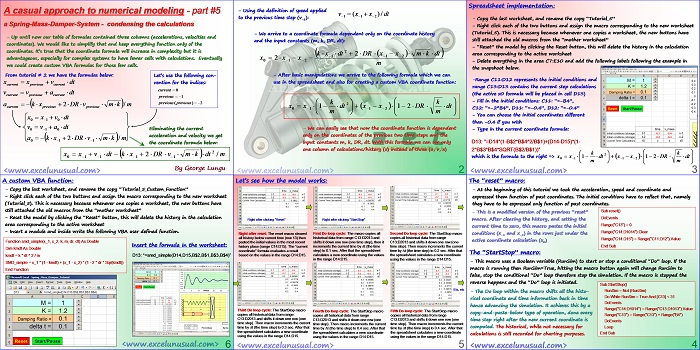# Casual Introduction to Numerical Methods – spring-mass-damper system model – part#5

In this tutorial, most of the calculations for the numerical simulation a SMD (spring-mas-damper) system will be consolidated into a single formula, the coordinate formula. In this case, in order to calculate the coordinate at the end of a any time step, we will need just the coordinates from the previous two time steps and of course the input parameters (constants). These input parameters are: mass, damping ratio, spring constant and time step. There will be no more need for velocity or acceleration computations. At the end of the presentation a simple user defined VBA function is demonstrated in a new worksheet.[sociallocker][/sociallocker]